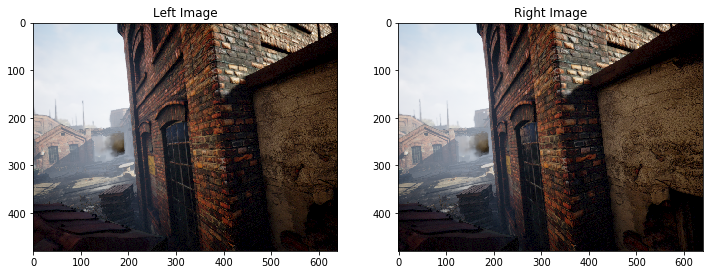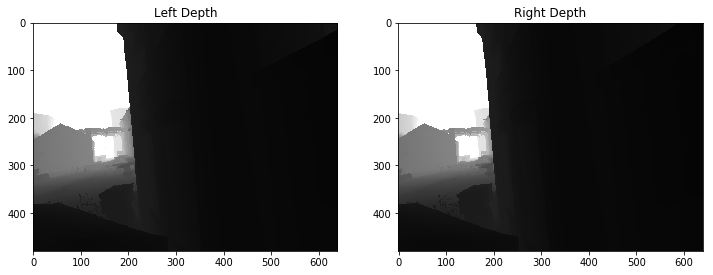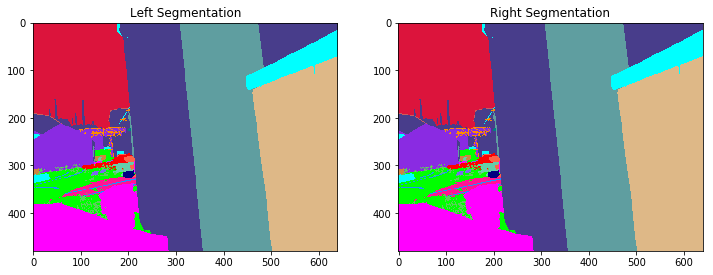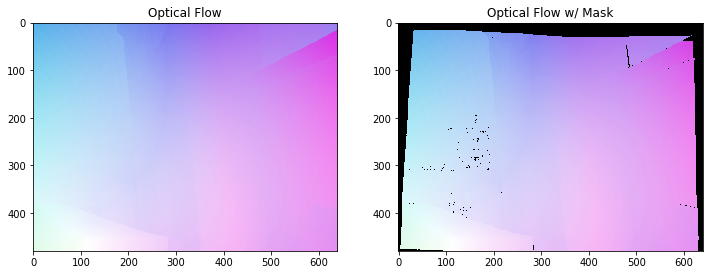# TartanAir: AirSim Simulation Dataset for Simultaneous Localization and Mapping

VSLAM AirSim Images Tartan Air

TartanAir AirSim Autonomous vehicle data generated to solve Simultaneous Localization and Mapping (SLAM)

Simultaneous Localization and Mapping (SLAM) is one of the most fundamental capabilities necessary for robots. Due to the ubiquitous availability of images, Visual SLAM (V-SLAM) has become an important component of many autonomous systems. Impressive progress has been made with both geometric-based methods and learning-based methods. However, developing robust and reliable SLAM methods for real-world applications is still a challenging problem. Real-life environments are full of difficult cases such as light changes or lack of illumination, dynamic objects, and texture-less scenes. This dataset takes advantages of the advancing computer graphics technology, and aims to cover diverse scenarios with challenging features in simulation.The data is collected in photo-realistic simulation environments in the presence of various light conditions, weather and moving objects. By collecting data in simulation, we are able to obtain multi-modal sensor data and precise ground truth labels, including the stereo RGB image, depth image, segmentation, optical flow, and camera poses. We set up a large number of environments with various styles and scenes, covering challenging viewpoints and diverse motion patterns, which are difficult to achieve by using physical data collection platforms. The four most important features of our dataset are: 1) Large size diverse realistic data; 2) Multimodal ground truth labels; 3) Diversity of motion patterns; 4) Challenging Scenes.

This dataset provides 5 types of data, including:

• Stereo images: image type (png).

• Depth file: numpy type (npy).

• Segmentation file: numpy type (npy).

• Optical flow file: numpy type (npy).

• Camera pose file: text type (txt).

It is collected from different environments, contains hundreds of trajectories (3TB) in total as of 2019.#### Challenging visual effects

In some simulations, The dataset simulates multiple types of challenging visual effects.

• Hard lighting conditions. Day-night alternating. Low-lighting. Rapidly changing illuminations.
• Weather effects. Clear, raining, snowing, windy, and fog.
• Seasonal change.#### Storage Location

This dataset is stored in the East US Azure region. Allocating compute resources in East US is recommended for affinity.

#### Citation

More technical details are available in AirSim paper (FSR 2017 Conference). Please cite this as:

```@article{tartanair2020arxiv, title = {TartanAir: A Dataset to Push the Limits of Visual SLAM}, author = {Wenshan Wang, Delong Zhu, Xiangwei Wang, Yaoyu Hu, Yuheng Qiu, Chen Wang, Yafei Hu, Ashish Kapoor, Sebastian Scherer}, journal = {arXiv preprint arXiv:2003.14338}, year = {2020}, url = {https://arxiv.org/abs/2003.14338} } @inproceedings{airsim2017fsr, author = {Shital Shah and Debadeepta Dey and Chris Lovett and Ashish Kapoor}, title = {AirSim: High-Fidelity Visual and Physical Simulation for Autonomous Vehicles}, year = {2017}, booktitle = {Field and Service Robotics}, eprint = {arXiv:1705.05065}, url = {https://arxiv.org/abs/1705.05065} }```

#### Contact

Email if you have any questions about the data source. You can also reach out to contributers on the associated GitHub.

#### Notices

MICROSOFT PROVIDES AZURE OPEN DATASETS ON AN “AS IS” BASIS. MICROSOFT MAKES NO WARRANTIES, EXPRESS OR IMPLIED, GUARANTEES OR CONDITIONS WITH RESPECT TO YOUR USE OF THE DATASETS. TO THE EXTENT PERMITTED UNDER YOUR LOCAL LAW, MICROSOFT DISCLAIMS ALL LIABILITY FOR ANY DAMAGES OR LOSSES, INCLUDING DIRECT, CONSEQUENTIAL, SPECIAL, INDIRECT, INCIDENTAL OR PUNITIVE, RESULTING FROM YOUR USE OF THE DATASETS.

This dataset is provided under the original terms that Microsoft received source data. The dataset may include data sourced from Microsoft.

### Access

Available inWhen to use
Azure Notebooks

Quickly explore the dataset with Jupyter notebooks hosted on Azure or your local machine.

Azure Notebooks

### Azure Notebooks

Package: Language: Python

## Demo notebook for accessing TartanAir data on Azure¶

!! NOTE: This sample file should only be used on Azure. To download the data to your local machine, please refer to the website: http://theairlab.org/tartanair-dataset/

This notebook provides an example of accessing TartanAir data from blobl storage on Azure, including:

1) navigate the directories of different environments and trajectories.

2) load the data into memory, and

3) visualize the data.

## Data directory structure¶

``````ROOT
|
--- ENV_NAME_0                             # environment folder
|       |
|       ---- Easy                          # difficulty level
|       |      |
|       |      ---- P000                   # trajectory folder
|       |      |      |
|       |      |      +--- depth_left      # 000000_left_depth.npy - 000xxx_left_depth.npy
|       |      |      +--- depth_right     # 000000_right_depth.npy - 000xxx_right_depth.npy
|       |      |      +--- image_left      # 000000_left.png - 000xxx_left.png
|       |      |      +--- image_right     # 000000_right.png - 000xxx_right.png
|       |      |      +--- seg_left        # 000000_left_seg.npy - 000xxx_left_seg.npy
|       |      |      +--- seg_right       # 000000_right_seg.npy - 000xxx_right_seg.npy
|       |      |      ---- pose_left.txt
|       |      |      ---- pose_right.txt
|       |      |
|       |      +--- P001
|       |      .
|       |      .
|       |      |
|       |      +--- P00K
|       |
|       +--- Hard
|
+-- ENV_NAME_1
.
.
|
+-- ENV_NAME_N``````

## Notebook dependencies¶

`pip install numpy`

`pip install azure-storage-blob`

`pip install opencv-python`

## Imports and contrainer_client¶

In :
```from azure.storage.blob import ContainerClient
import numpy as np
import io
import cv2
import time
import matplotlib.pyplot as plt
%matplotlib inline

# Dataset website: http://theairlab.org/tartanair-dataset/
account_url = 'https://tartanair.blob.core.windows.net/'
container_name = 'tartanair-release1'

container_client = ContainerClient(account_url=account_url,
container_name=container_name,
credential=None)
```

## List the environments and trajectories¶

In :
```def get_environment_list():
'''
List all the environments shown in the root directory
'''
env_gen = container_client.walk_blobs()
envlist = []
for env in env_gen:
envlist.append(env.name)
return envlist

def get_trajectory_list(envname, easy_hard = 'Easy'):
'''
List all the trajectory folders, which is named as 'P0XX'
'''
assert(easy_hard=='Easy' or easy_hard=='Hard')
traj_gen = container_client.walk_blobs(name_starts_with=envname + '/' + easy_hard+'/')
trajlist = []
for traj in traj_gen:
trajname = traj.name
trajname_split = trajname.split('/')
trajname_split = [tt for tt in trajname_split if len(tt)>0]
if trajname_split[-1] == 'P':
trajlist.append(trajname)
return trajlist

def _list_blobs_in_folder(folder_name):
"""
List all blobs in a virtual folder in an Azure blob container
"""

files = []
generator = container_client.list_blobs(name_starts_with=folder_name)
for blob in generator:
files.append(blob.name)
return files

def get_image_list(trajdir, left_right = 'left'):
assert(left_right == 'left' or left_right == 'right')
files = _list_blobs_in_folder(trajdir + '/image_' + left_right + '/')
files = [fn for fn in files if fn.endswith('.png')]
return files

def get_depth_list(trajdir, left_right = 'left'):
assert(left_right == 'left' or left_right == 'right')
files = _list_blobs_in_folder(trajdir + '/depth_' + left_right + '/')
files = [fn for fn in files if fn.endswith('.npy')]
return files

def get_flow_list(trajdir, ):
files = _list_blobs_in_folder(trajdir + '/flow/')
files = [fn for fn in files if fn.endswith('flow.npy')]
return files

files = _list_blobs_in_folder(trajdir + '/flow/')
files = [fn for fn in files if fn.endswith('mask.npy')]
return files

def get_posefile(trajdir, left_right = 'left'):
assert(left_right == 'left' or left_right == 'right')
return trajdir + '/pose_' + left_right + '.txt'

def get_seg_list(trajdir, left_right = 'left'):
assert(left_right == 'left' or left_right == 'right')
files = _list_blobs_in_folder(trajdir + '/seg_' + left_right + '/')
files = [fn for fn in files if fn.endswith('.npy')]
return files
```

### List all the environments¶

In :
```envlist = get_environment_list()
print('Find {} environments..'.format(len(envlist)))
print(envlist)
```
```Find 18 environments..
['abandonedfactory/', 'abandonedfactory_night/', 'amusement/', 'carwelding/', 'endofworld/', 'gascola/', 'hospital/', 'japanesealley/', 'neighborhood/', 'ocean/', 'office/', 'office2/', 'oldtown/', 'seasidetown/', 'seasonsforest/', 'seasonsforest_winter/', 'soulcity/', 'westerndesert/']
```

### List all the 'Easy' trajectories in the first environment¶

In :
```diff_level = 'Easy'
env_ind = 0
trajlist = get_trajectory_list(envlist[env_ind], easy_hard = diff_level)
print('Find {} trajectories in {}'.format(len(trajlist), envlist[env_ind]+diff_level))
print(trajlist)
```
```Find 10 trajectories in abandonedfactory/Easy
['abandonedfactory/Easy/P000/', 'abandonedfactory/Easy/P001/', 'abandonedfactory/Easy/P002/', 'abandonedfactory/Easy/P004/', 'abandonedfactory/Easy/P005/', 'abandonedfactory/Easy/P006/', 'abandonedfactory/Easy/P008/', 'abandonedfactory/Easy/P009/', 'abandonedfactory/Easy/P010/', 'abandonedfactory/Easy/P011/']
```

### List all the data files in one trajectory¶

In :
```traj_ind = 1
traj_dir = trajlist[traj_ind]

left_img_list = get_image_list(traj_dir, left_right = 'left')
print('Find {} left images in {}'.format(len(left_img_list), traj_dir))

right_img_list = get_image_list(traj_dir, left_right = 'right')
print('Find {} right images in {}'.format(len(right_img_list), traj_dir))

left_depth_list = get_depth_list(traj_dir, left_right = 'left')
print('Find {} left depth files in {}'.format(len(left_depth_list), traj_dir))

right_depth_list = get_depth_list(traj_dir, left_right = 'right')
print('Find {} right depth files in {}'.format(len(right_depth_list), traj_dir))

left_seg_list = get_seg_list(traj_dir, left_right = 'left')
print('Find {} left segmentation files in {}'.format(len(left_seg_list), traj_dir))

right_seg_list = get_seg_list(traj_dir, left_right = 'left')
print('Find {} right segmentation files in {}'.format(len(right_seg_list), traj_dir))

flow_list = get_flow_list(traj_dir)
print('Find {} flow files in {}'.format(len(flow_list), traj_dir))

left_pose_file = get_posefile(traj_dir, left_right = 'left')
print('Left pose file: {}'.format(left_pose_file))

right_pose_file = get_posefile(traj_dir, left_right = 'right')
print('Right pose file: {}'.format(right_pose_file))
```
```Find 434 left images in abandonedfactory/Easy/P001/
Find 434 right images in abandonedfactory/Easy/P001/
Find 434 left depth files in abandonedfactory/Easy/P001/
Find 434 right depth files in abandonedfactory/Easy/P001/
Find 434 left segmentation files in abandonedfactory/Easy/P001/
Find 434 right segmentation files in abandonedfactory/Easy/P001/
Find 433 flow files in abandonedfactory/Easy/P001/
Find 433 flow mask files in abandonedfactory/Easy/P001/
Left pose file: abandonedfactory/Easy/P001//pose_left.txt
Right pose file: abandonedfactory/Easy/P001//pose_right.txt
```

In :
```def read_numpy_file(numpy_file,):
'''
return a numpy array given the file path
'''
bc = container_client.get_blob_client(blob=numpy_file)
ee = io.BytesIO(data.content_as_bytes())
return ff

'''
return a uint8 numpy array given the file path
'''
bc = container_client.get_blob_client(blob=image_file)
ee = io.BytesIO(data.content_as_bytes())
im_rgb = img[:, :, [2, 1, 0]] # BGR2RGB
return im_rgb
```

## Functions for data visualization¶

In :
```def depth2vis(depth, maxthresh = 50):
depthvis = np.clip(depth,0,maxthresh)
depthvis = depthvis/maxthresh*255
depthvis = depthvis.astype(np.uint8)
depthvis = np.tile(depthvis.reshape(depthvis.shape+(1,)), (1,1,3))

return depthvis

def seg2vis(segnp):
colors = [(205, 92, 92), (0, 255, 0), (199, 21, 133), (32, 178, 170), (233, 150, 122), (0, 0, 255), (128, 0, 0), (255, 0, 0), (255, 0, 255), (176, 196, 222), (139, 0, 139), (102, 205, 170), (128, 0, 128), (0, 255, 255), (0, 255, 255), (127, 255, 212), (222, 184, 135), (128, 128, 0), (255, 99, 71), (0, 128, 0), (218, 165, 32), (100, 149, 237), (30, 144, 255), (255, 0, 255), (112, 128, 144), (72, 61, 139), (165, 42, 42), (0, 128, 128), (255, 255, 0), (255, 182, 193), (107, 142, 35), (0, 0, 128), (135, 206, 235), (128, 0, 0), (0, 0, 255), (160, 82, 45), (0, 128, 128), (128, 128, 0), (25, 25, 112), (255, 215, 0), (154, 205, 50), (205, 133, 63), (255, 140, 0), (220, 20, 60), (255, 20, 147), (95, 158, 160), (138, 43, 226), (127, 255, 0), (123, 104, 238), (255, 160, 122), (92, 205, 92),]
segvis = np.zeros(segnp.shape+(3,), dtype=np.uint8)

for k in range(256):
colorind = k % len(colors)

return segvis

def _calculate_angle_distance_from_du_dv(du, dv, flagDegree=False):
a = np.arctan2( dv, du )

angleShift = np.pi

if ( True == flagDegree ):
a = a / np.pi * 180
angleShift = 180
# print("Convert angle from radian to degree as demanded by the input file.")

d = np.sqrt( du * du + dv * dv )

return a, d, angleShift

def flow2vis(flownp, maxF=500.0, n=8, mask=None, hueMax=179, angShift=0.0):
"""
Show a optical flow field as the KITTI dataset does.
Some parts of this function is the transform of the original MATLAB code flow_to_color.m.
"""

ang, mag, _ = _calculate_angle_distance_from_du_dv( flownp[:, :, 0], flownp[:, :, 1], flagDegree=False )

# Use Hue, Saturation, Value colour model
hsv = np.zeros( ( ang.shape, ang.shape, 3 ) , dtype=np.float32)

am = ang < 0
ang[am] = ang[am] + np.pi * 2

hsv[ :, :, 0 ] = np.remainder( ( ang + angShift ) / (2*np.pi), 1 )
hsv[ :, :, 1 ] = mag / maxF * n
hsv[ :, :, 2 ] = (n - hsv[:, :, 1])/n

hsv[:, :, 0] = np.clip( hsv[:, :, 0], 0, 1 ) * hueMax
hsv[:, :, 1:3] = np.clip( hsv[:, :, 1:3], 0, 1 ) * 255
hsv = hsv.astype(np.uint8)

rgb = cv2.cvtColor(hsv, cv2.COLOR_HSV2RGB)

if ( mask is not None ):
rgb[mask] = np.array([0, 0 ,0], dtype=np.uint8)

return rgb
```

In :
```data_ind = 173 # randomly select one frame (data_ind < TRAJ_LEN)
```

#### Visualize the left and right RGB images¶

In :
```left_img = read_image_file(left_img_list[data_ind])

plt.figure(figsize=(12, 5))
plt.subplot(121)
plt.imshow(left_img)
plt.title('Left Image')
plt.subplot(122)
plt.imshow(right_img)
plt.title('Right Image')
plt.show()
```#### Visualize the left and right depth files¶

In :
```left_depth = read_numpy_file(left_depth_list[data_ind])
left_depth_vis = depth2vis(left_depth)

right_depth_vis = depth2vis(right_depth)

plt.figure(figsize=(12, 5))
plt.subplot(121)
plt.imshow(left_depth_vis)
plt.title('Left Depth')
plt.subplot(122)
plt.imshow(right_depth_vis)
plt.title('Right Depth')
plt.show()
```#### Visualize the left and right segmentation files¶

In :
```left_seg = read_numpy_file(left_seg_list[data_ind])
left_seg_vis = seg2vis(left_seg)

right_seg_vis = seg2vis(right_seg)

plt.figure(figsize=(12, 5))
plt.subplot(121)
plt.imshow(left_seg_vis)
plt.title('Left Segmentation')
plt.subplot(122)
plt.imshow(right_seg_vis)
plt.title('Right Segmentation')
plt.show()
```#### Visualize the flow and mask files¶

In :
```flow = read_numpy_file(flow_list[data_ind])
flow_vis = flow2vis(flow)